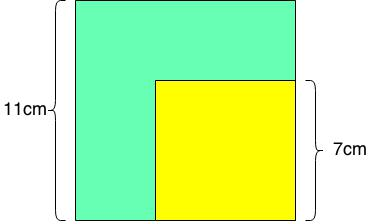# Order of operations with exponents

### Order of operations with exponents

When you are solving questions with exponents, you must keep in mind that order of operation (BEDMAS/PEDMAS) still applies. In this lesson, we will get you familiar with how BEDMAS/PEDMAS works with exponents.

#### Lessons

• Introduction
a)
Powers VS. Exponents

• 1.
Calculate:
a)
$5{\left( { - 2} \right)^4}$

b)
$- 6\left( {{3^7}} \right)$

c)
$8{\left( 4 \right)^3}$

d)
$9\left( { - {4^2}} \right)$

• 2.
Calculate:
a)
$30 - {5^2} + \left( {{6^3} - {2^3}} \right)$

b)
$9 - 4\left( {{2^3}} \right)$

c)
${\left( { - 5 - 4} \right)^2} + {\left( { - 4} \right)^2}$

d)
${\left( { - 4} \right)^6} \div {2^3}$

• 3.
A small yellow square is put on top of a large green square. The side length of the yellow square is 7 cm, whereas the side length of the green square is 11 cm.a)
Write the visible green area as powers.

b)
Calculate the visible green area.